Скачать презентацию 5 -4 Writing a Function Rule This lesson

9c394e5e21f1df77f22585783d0e0d87.ppt

• Количество слайдов: 9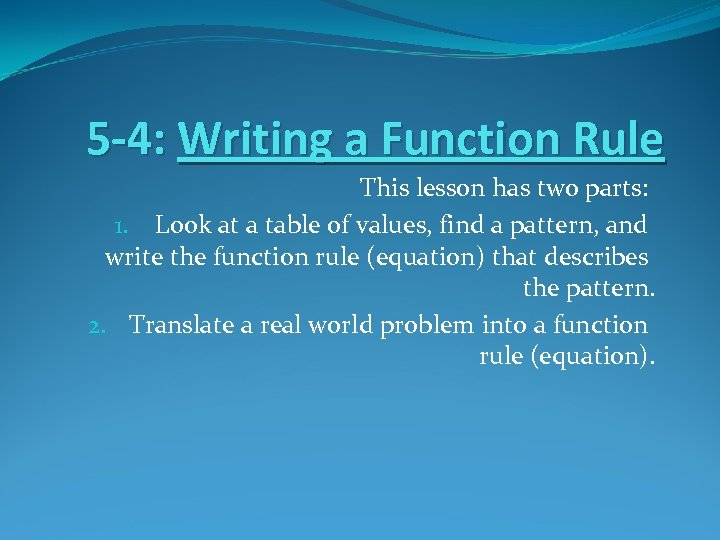5 -4: Writing a Function Rule This lesson has two parts: 1. Look at a table of values, find a pattern, and write the function rule (equation) that describes the pattern. 2. Translate a real world problem into a function rule (equation).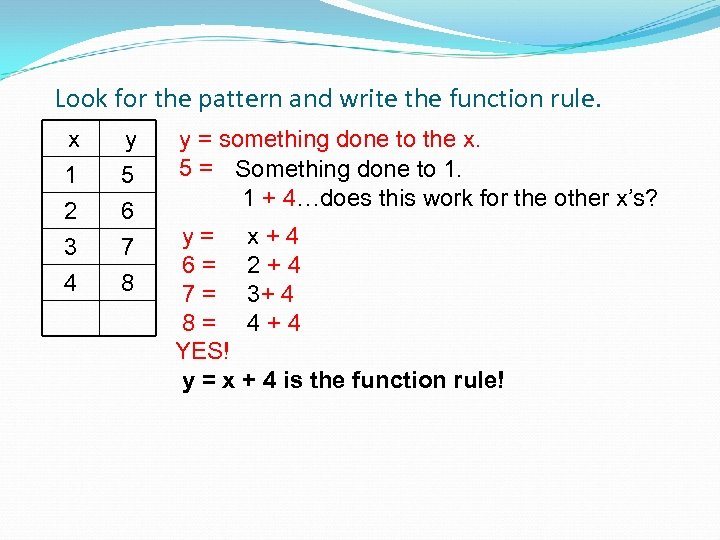Look for the pattern and write the function rule. x 1 2 3 y 5 6 7 4 8 y = something done to the x. 5 = Something done to 1. 1 + 4…does this work for the other x’s? y = x + 4 6 = 2 + 4 7 = 3+ 4 8 = 4 + 4 YES! y = x + 4 is the function rule!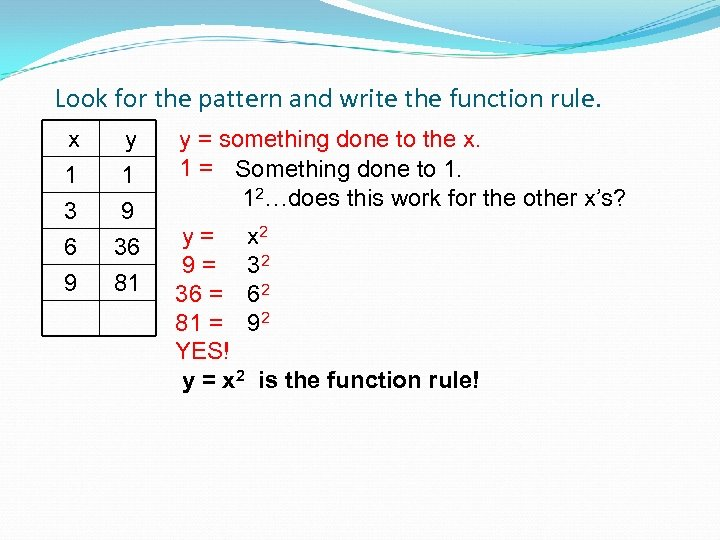Look for the pattern and write the function rule. x 1 3 6 y 1 9 36 9 81 y = something done to the x. 1 = Something done to 1. 12…does this work for the other x’s? y = x 2 9 = 32 36 = 62 81 = 92 YES! y = x 2 is the function rule!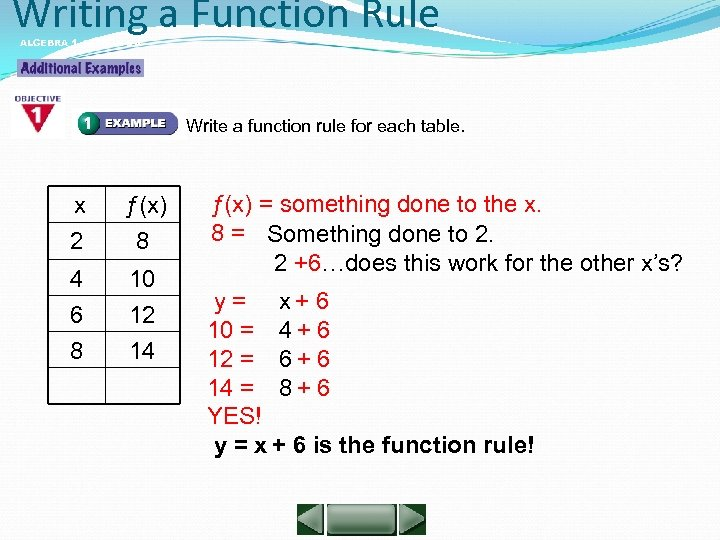Writing a Function Rule ALGEBRA 1 LESSON 5 -4 Write a function rule for each table. x 2 ƒ(x) 8 4 6 10 12 8 14 ƒ(x) = something done to the x. 8 = Something done to 2. 2 +6…does this work for the other x’s? y = x + 6 10 = 4 + 6 12 = 6 + 6 14 = 8 + 6 YES! y = x + 6 is the function rule!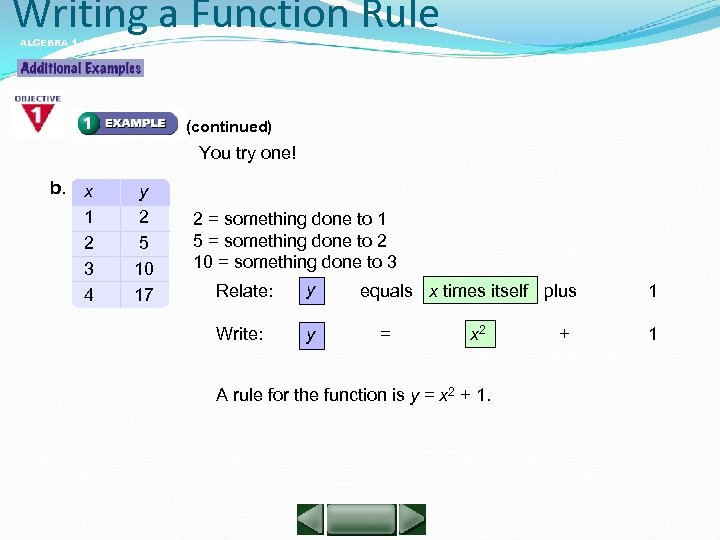Writing a Function Rule ALGEBRA 1 LESSON 5 -4 (continued) You try one! b. x 1 2 3 4 y 2 5 10 17 2 = something done to 1 5 = something done to 2 10 = something done to 3 Relate: y equals x times itself plus Write: y = x 2 + A rule for the function is y = x 2 + 1. 1 1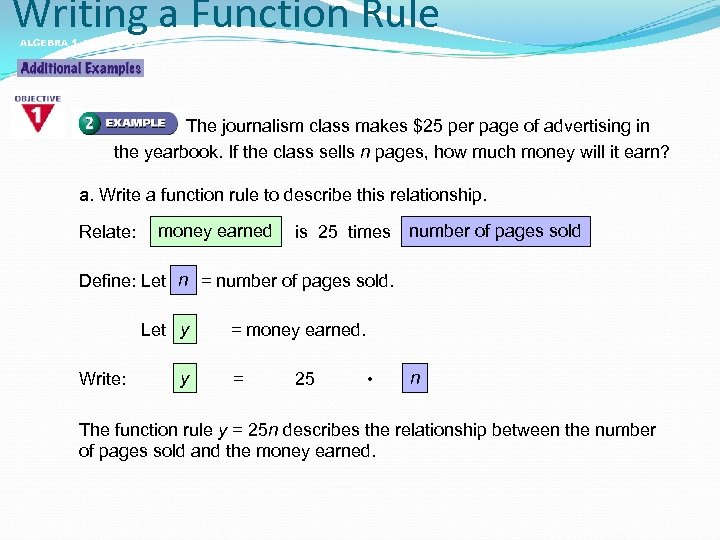Writing a Function Rule ALGEBRA 1 LESSON 5 -4 The journalism class makes \$25 per page of advertising in the yearbook. If the class sells n pages, how much money will it earn? a. Write a function rule to describe this relationship. Relate: money earned is 25 times number of pages sold n Define: Let = number of pages sold. y Let = money earned. Write: y = 25 • n The function rule y = 25 n describes the relationship between the number of pages sold and the money earned.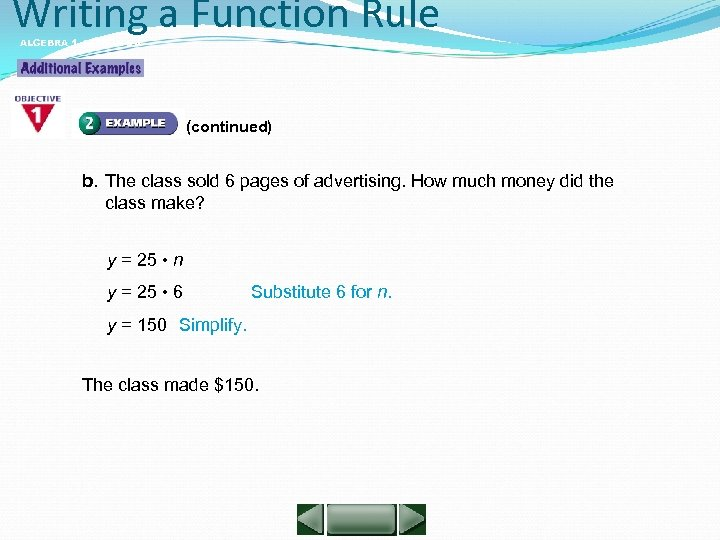Writing a Function Rule ALGEBRA 1 LESSON 5 -4 (continued) b. The class sold 6 pages of advertising. How much money did the class make? y = 25 • n y = 25 • 6 Substitute 6 for n. y = 150 Simplify. The class made \$150.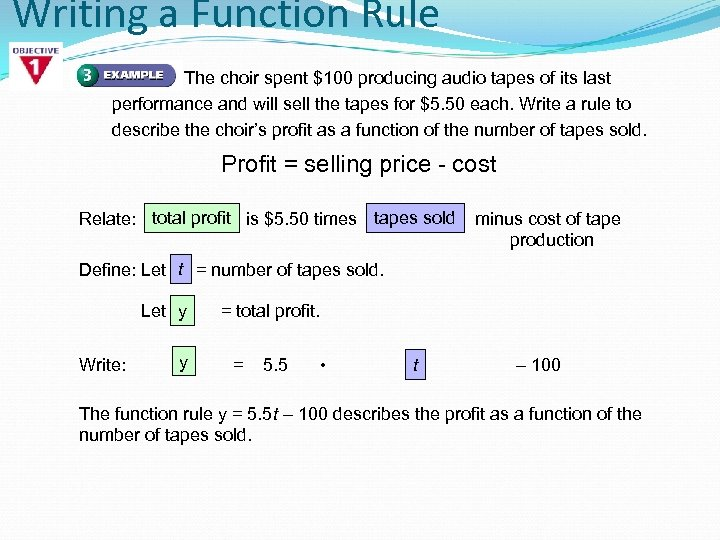Writing a Function Rule The choir spent \$100 producing audio tapes of its last performance and will sell the tapes for \$5. 50 each. Write a rule to describe the choir’s profit as a function of the number of tapes sold. Profit = selling price - cost Relate: total profit is \$5. 50 times tapes sold minus cost of tape production t Define: Let = number of tapes sold. y Let = total profit. Write: y = 5. 5 • t – 100 The function rule y = 5. 5 t – 100 describes the profit as a function of the number of tapes sold.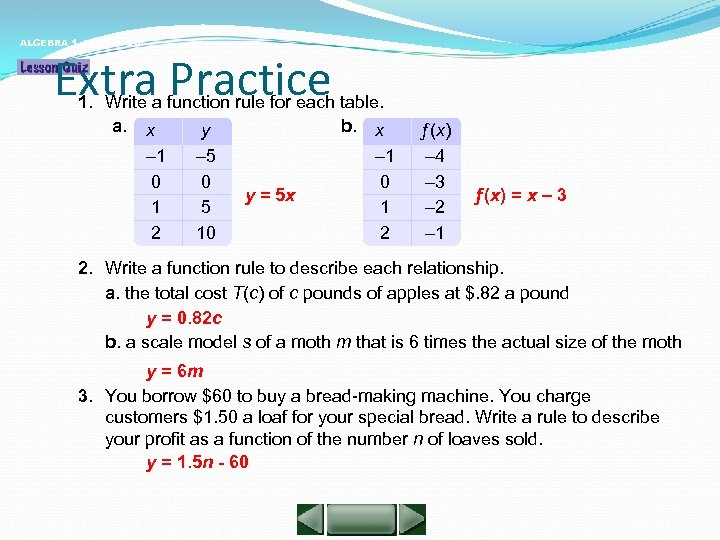ALGEBRA 1 LESSON 5 -4 Extra Practice 1. Write a function rule for each table. a. x b. x y – 1 0 1 2 – 5 0 5 10 y = 5 x – 1 0 1 2 ƒ(x) – 4 – 3 – 2 – 1 ƒ(x) = x – 3 2. Write a function rule to describe each relationship. a. the total cost T(c) of c pounds of apples at \$. 82 a pound y = 0. 82 c b. a scale model s of a moth m that is 6 times the actual size of the moth y = 6 m 3. You borrow \$60 to buy a bread-making machine. You charge customers \$1. 50 a loaf for your special bread. Write a rule to describe your profit as a function of the number n of loaves sold. y = 1. 5 n - 60